# Curvy Equation

##### Age 16 to 18Challenge Level

Siddhant from Singapore International School in India and Wiktor and Jiali from LAE Tottenham in the UK sketched the function correctly.

Jiali found the $x$-intercept: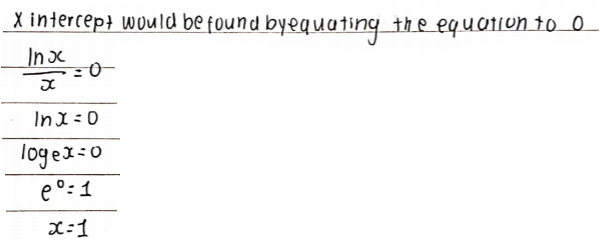Siddhant wrote:
At $x=0,$ the $\dfrac{\ln{x}}{x}$ is undefined, but $0$ is not in the domain of $\text{h}(x)$

To investigate what happens as $x\rightarrow0$, Jiali substituted in some values of $x.$ Jiali also substituted in larger values of $x$ to investigate the shape of the curve: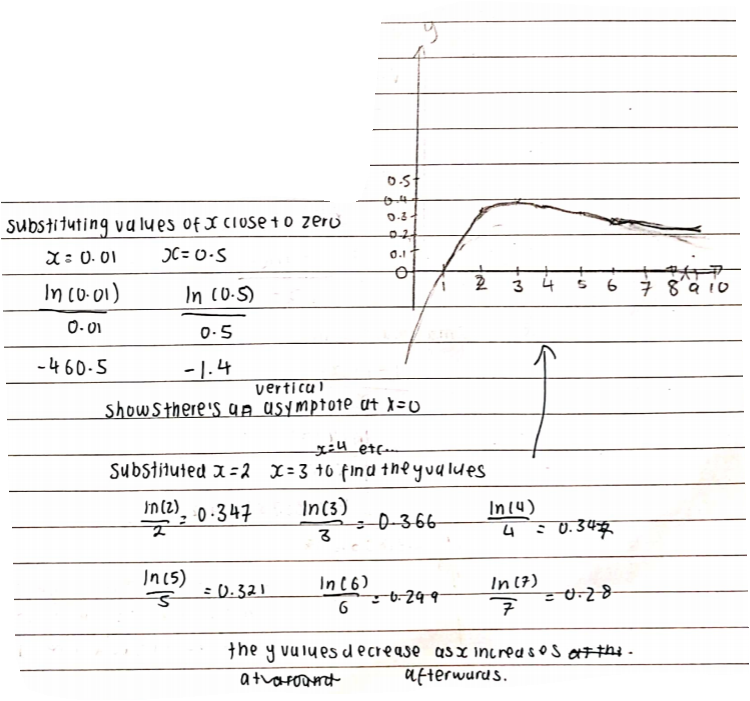Wiktor described what happens for large values of $x,$ using the graphs of $y=x$ and $y=\ln{x}$ to help: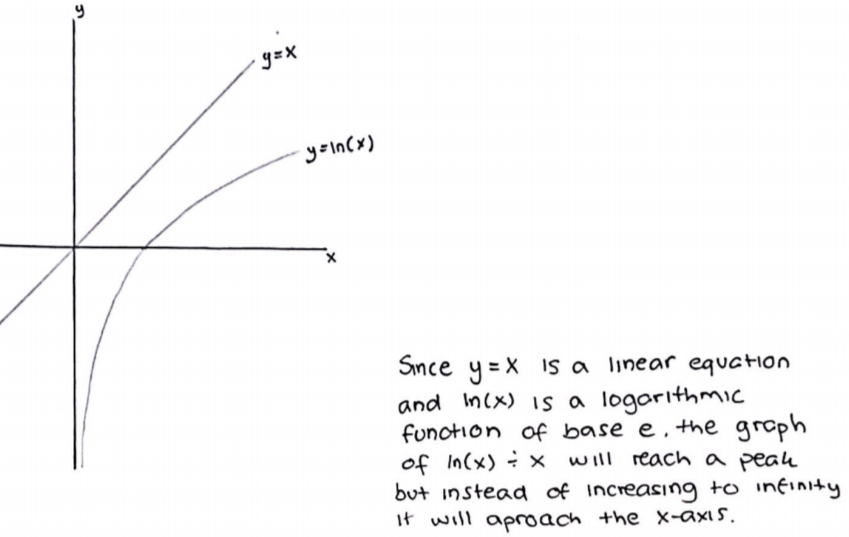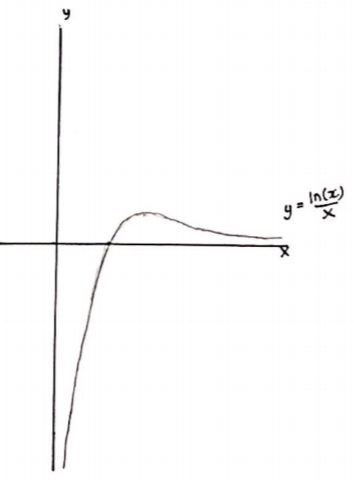Siddhant, Wiktor and Jiali all differntiated $\text{h}(x)$ using the quotient rule and Siddhant used $\text{h}'(x)$ to understand the shape of the graph. Siddhant wrote:
\begin{align}\text{h}'(x) &= \frac{\frac{\text{d}}{\text{d}x}\left[\ln{x}\right].x - \ln{x}.\frac{\text{d}}{\text{d}x}\left[x\right]}{x^2}\\ &=\frac{1-\ln{x}}{x^2}\end{align} Stationary point:
$\dfrac{1-\ln{x}}{x^2} = 0\\ 1-\ln{x}=0\\ \ln{x}=1\\ x=e$

Therefore $\text{h}(x)$ is stationary at $x=e$

$\rightarrow$ Therefore as $x$ approaches $e,$ $\text{h}(x)$ increases
$\rightarrow$ As $x$ increases from $e,$ $\text{h}(x)$ decreases because $\text{h}'(x)$ becomes negative (and so must have a horizontal asymptote since Jiali showed it doesn't cross the $x$ axis again)

Siddhant, Wiktor and Jiali used the graph to solve the equation $n^m=m^n$. Jiali began: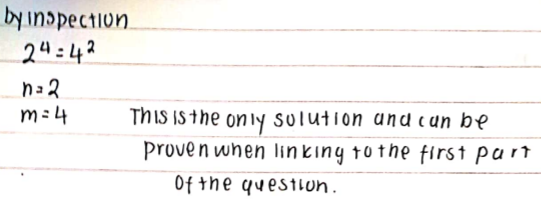Siddhant showed how the equation can be related to the graph:
$n^m=m^n$
Take $\ln$ on both sides \begin{align} \ln{n^m} &= \ln{m^n}\\ m\ln{n} &= n\ln{m} \\ \frac{\ln{n}}n &=\frac{\ln{m}}m\end{align} We know:
$\text{h}(m) = \dfrac{\ln{m}}{m}\\ \text{h}(n) = \dfrac{\ln{n}}{n}$

Therefore, $n^m = m^n$ can be written as $\text{h}(m) = \text{h}(n)$

Using the graph, $\text{h}(x)$ is many-one after $x=1$ and is stationary at $x=e$.

Jiali and Wiktor showed this graphically. This is Wiktor's work: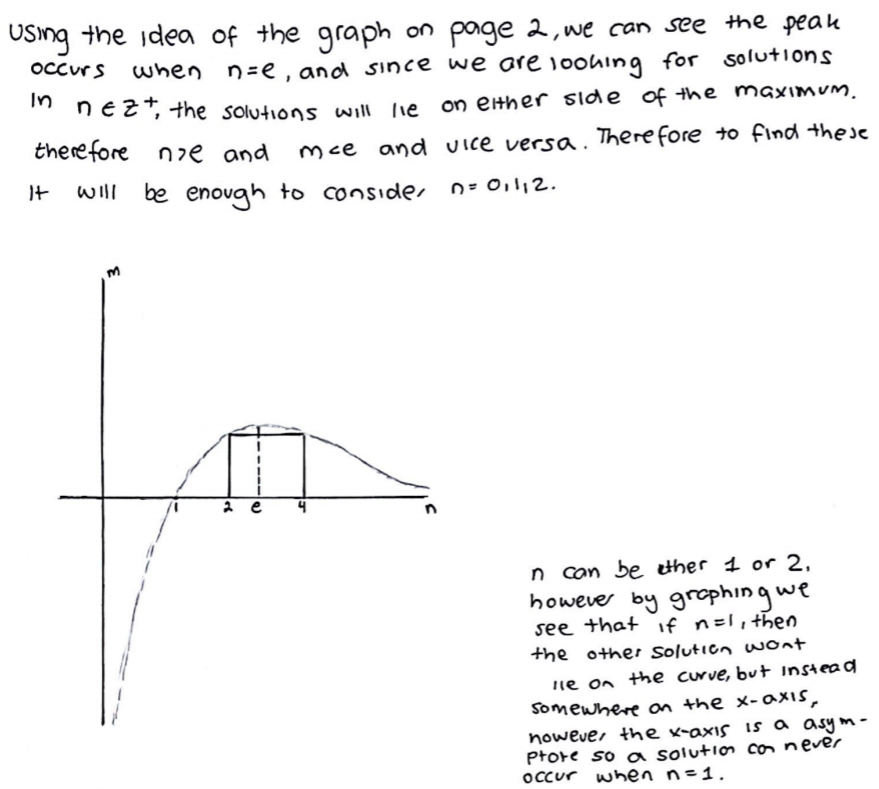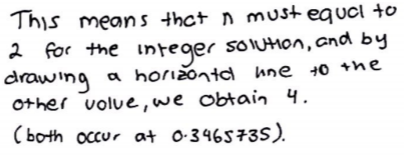Wiktor then substituted $2$ and $4$ into the equation to check that they work. Siddhant found $4$ by trial.

Well done!